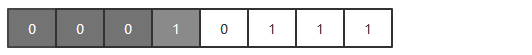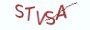# 2.5.1 问题说明# 2.5.3 算法实现

```# __author__ = 薯条老师
import random

def calc_max4b(n):
"""
:param n: 十进制整数
:return: 返回最大的四位值
"""

base, max4b = 16, 0
n = -n if n < 0 else n
# 按除16取余，再逆序排列的算法来计算十进制的每一个十六进制位
while n >= 1:
h, n = n % base,  n // base
if h > max4b:
max4b = h
return max4b

if __name__ == "__main__":
number = random.randint(0,  10**4)
print("{}的最大四位值是:{}".format(number, calc_max4b(number)))```

# 2.5.4 最具实力的小班培训

`(1) Python后端工程师高薪就业班，月薪11K-18K，免费领取课程大纲(2) Python爬虫工程师高薪就业班，年薪十五万，免费领取课程大纲(3) Java后端开发工程师高薪就业班，月薪11K-20K, 免费领取课程大纲(4) Python大数据分析，量化投资就业班，月薪12K-25K,免费领取课程大纲`## 注册账号